# Laws of Motion and friction Numericals

Question 1
A body of Mass m moves along the X-axis such that a time t its position is given by following expression $x=at^{3/2} -bt + c$ . Where a, b and c are constant
(a) Calculate the acceleration of the body
(b) What is the force acting on it
(c) What is the force at t=1 sec
(d) What are the dimension of a

Question 2
An 8 Kg object is subjected to three forces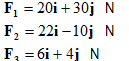(a) Find the acceleration of the object.
(b) If the object starts from rest from origin, what will be the location after 4 sec
(c) What is the magnitude of resultant force and its direction?
(d) What fourth force F4 should be applied on the body to make the net resultant force zero

Question 3
Consider a three body system shown in figure below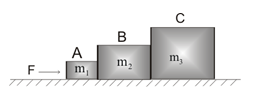(a) Find the acceleration of the each object
(b) Find the contact force between all the objects

Question 4
Three Block of mass m1, m2  and m3 are connected as shown in the figure below.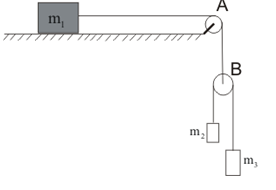All the surfaces are frictionless and strings and pulley are light. Find the acceleration of all the masses

Question 5
A small block of mass m1= 3 kg  is placed at rest on a larger block of mass m2=5 kg .The situation is given in the figure below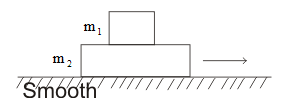The coefficient of friction between the two block  is µ=.3
And the horizontal surface is smooth.A constant Force F is applied on the block
Find out the following
(a) Find the value of limiting friction between the two blocks
(b) What is the maximum acceleration  by which the upper block can move
(c) What is the maximum value of F at which  both the block move together
(d) if F=20 N,what is the acceleration of each block and what frictional force is acting between the block
(e) What is the normal contact force between the blocks
(f) what is the normal contact force between the larger block and the smooth surface
(g) if F=40 N,what is the acceleration of each block and what frictional force is acting between the block
(h) If the force is applied to the upper block,what will be the minimum force required so that there is relative motion between the block
Given  g=10 m/sec2

Question 6
A object of mass M is standing in a stationary lift. What pressure force N exerted by the object on the floor of the lift
(a)If the lift is stationary
(b) if the lift is moving upward with acceleration a
(c) if the lift is moving downward with acceleration a
(d) if the lift is falling freely
(e) if the lift is moving upward with constant velocity
(f) if the lift is moving downward with constant velocity

Question 7
A piece of uniform strings hangs vertically so that its free end just touches the horizontal surface of the table.The upper end of the strings is now released. Show that at any instant during the falling of string, the total force on the surface of the table is three times the weight of the part of the string lying on the surface

Question 8
Three blocks A, B,C are such as
M1=2 kg , M2=3 kg , M3=6 kg
They are connected as shown in the below figure.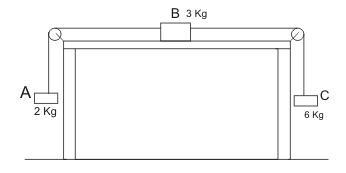The coefficient of friction between the block M2 and table is 0.2 Find out the following
(a) Draw all the forces acting on the system
(b) The acceleration of the system
(c) Frictional force between the block M2 and table
(d) Tension in the cord on the left and tension in the cord on the right
Given g=10 m/sec2
The pulley are light and friction less

Question 9
A boxcar is moving such that   Initial velocity v=0
And Acceleration= (4 m/sec2 ) i. Two objects A and B of mass 2 kg is kept in the boxcar. Take g=10m/sec2
Find out following
(a) If the object A slid along the frictionless floor with the velocity v= (10m/s) i. Find out the equation of the motion of object from the frame of reference of Boxcar. Also what time would it take to reach it original position relative to box car
(b) The object B slid along the rough floor with the velocity v= (10m/s) i. Find out the equation of the motion of object from the frame of reference of Boxcar. Coefficient of sliding friction =.3, Coefficient of Static friction >0.5

Question 10
A bead of mass M is fitted  onto a rod with a length of 2s and can move it without any friction. At t=0,the bead is in the middle of the  rod. The rod moves translationally in a horizontal plane with an acceleration a in a direction forming an angle α with the rod.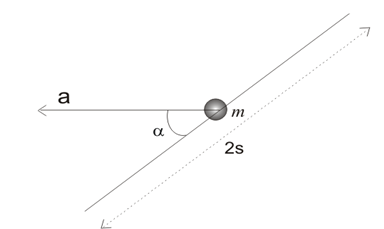(a) Find the acceleration of the bead relative to ground
(b) Find the acceleration of the bead relative to rod
(c) The reaction force exerted by the rod on the bead
(d) The time when the bead will leave the rod

Question 11
A mass less rod of length L with a small load (A) of mass m at the end is hinged at point X. And it is strictly vertically position touching a body B of mass M. A light jerk sets the system in motion. Let its separate out at angle α from the horizontal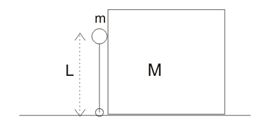Let i and j are the unit vectors across the X and Y axis
(a) what will be the velocity vectors of A and B at separation
(b) What will be centripetal acceleration of object A at time of separation?
(c) Find out the ratio of M/m in terms angle of separation
(d) If they separate out at angle α=30, what will the ratio of M/m
(e) What will be the velocity of A and B if α=30
(f) What will be value of tangential acceleration at separation?